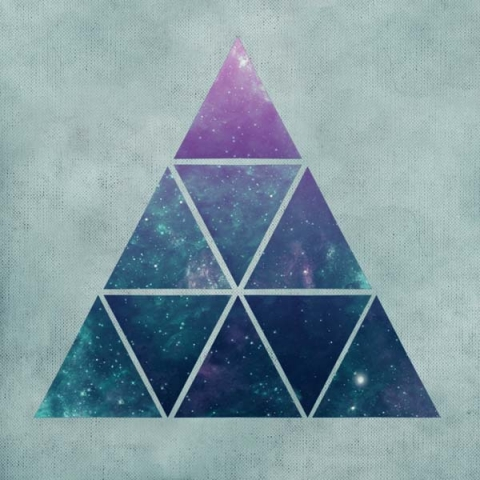As Carl Jung said, “Every tension of opposites culminates in a release, out of which comes the ‘third’. In the third, the tension is resolved and the lost unity is restored.”

# The Triad: Threeness: Harmony: Mediation

“All was divided into three.” Homer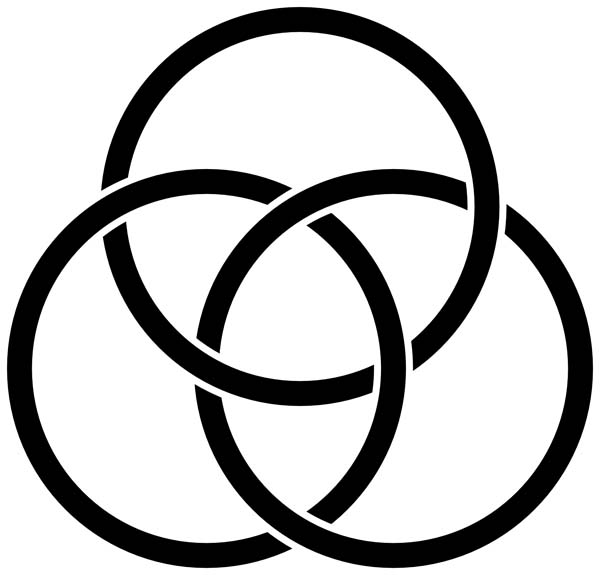# The Fundamental Principles of the Triad

Robert Lawlor wrote, “All things being dual by nature are three by principle.”

One and Two are considered parents to all the other numbers.

Three is the first and eldest child.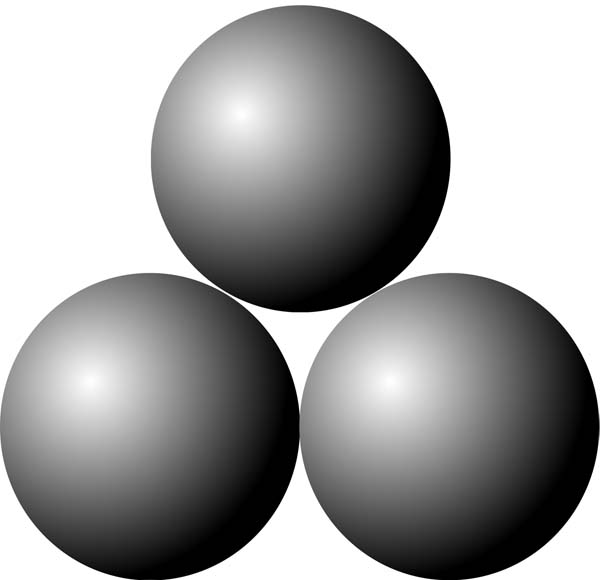Three pierces polarity by offering a way through to transcend opposites.

It shows the way to infinite possibilities where there had only been two before.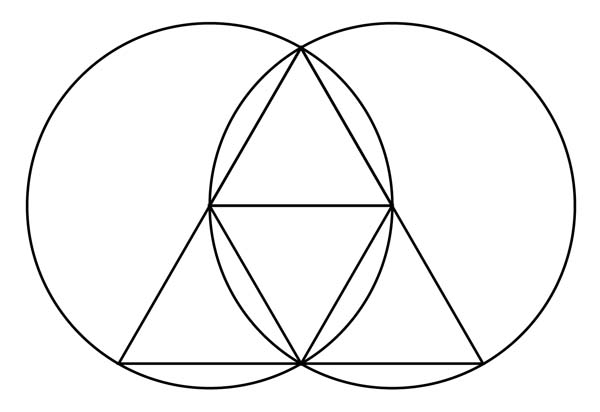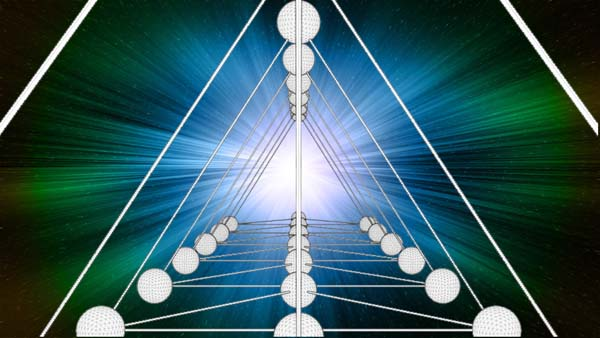It symbolizes rebirth, transformation and success and represents harmony, balance and divinity.

Threeis related to words like ‘through’; ‘threshold’ and the prefix ‘trans’ – meaning ‘across’ or ‘penetrate’.

The Triad takes us over a threshold and past polarized limits of the Dyad.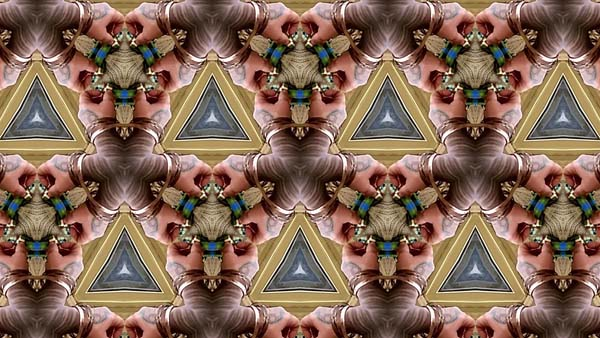Three according to the Nez Perce and Shahaptian Native American languages means “Center of the One.”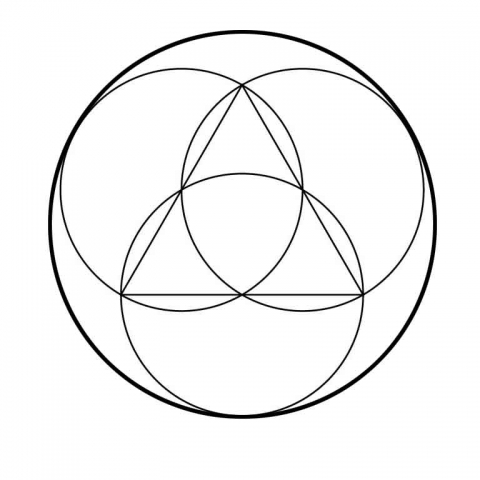######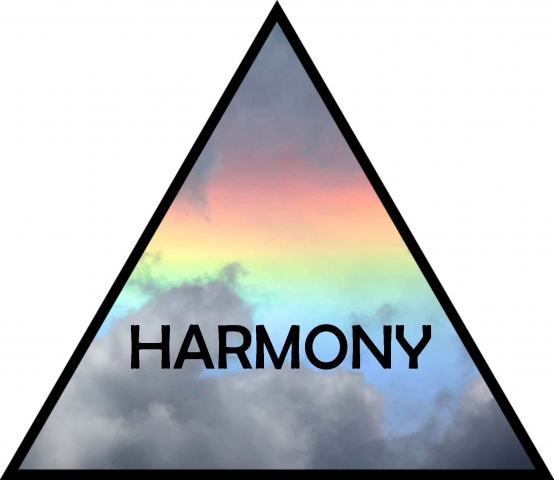“By harmony,” writes Gyorgy Doczi, “we generally mean a fitting, orderly and pleasant jointing of diversities, which in themselves may harbor many contrasts.  In this sense, harmony is a dinergic relationship, in which different and often contrasting elements complement each other by joining.”

Also:

• prudence
• wisdom
• piety
• friendship
• peace
• harmony
• unanimity
• marriage

### Atomic Number 3 = Lithium

Lithium is the lightest metal. It has the lowest density of any metal and can float on water. Lithium burns with a bright red flame. It is added to fireworks to make red sparks.

### Atomic Number 30 = Zinc

Zinc is the 24th most common element in the planet’s crust. It is a vital nutrient for both plants and animals.

### Atomic Number 33 = Arsenic

Arsenic occurs in three distinct solid forms. It is toxic to human and animal life.

# Arithmetic Properties of the Triad

Three (3) is the first odd masculine number.

Divisors: 1, 3

Three is the only number to equal the sum of all terms below it.

(3 = 2 + 1)

It is also the only number whose sum with those below it equals their product.

(1 + 2 + 3 = 1 x 2 x 3)

Three is a rough approximation of pi: (3.1415…)

It is the first odd prime number, second smallest prime, and the only prime triangular number.

3 is the fourth Fibonacci number.

It is the smallest number of sides that a simple polygon can have: a triangle.

Three of the 5 Platonic solids have triangular faces:

Tetrahedron, octahedron, icosahedron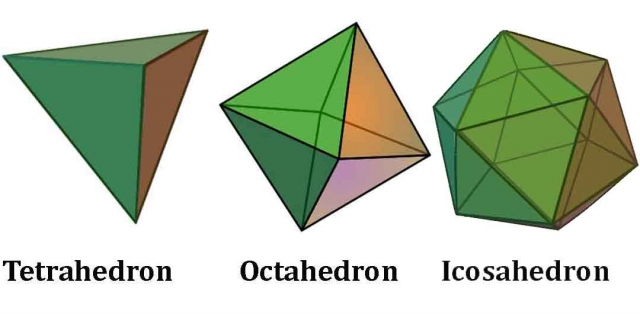Three of the 5 Platonic solids have vertices where three faces meet:

Tetrahedron, cube, dodecahedron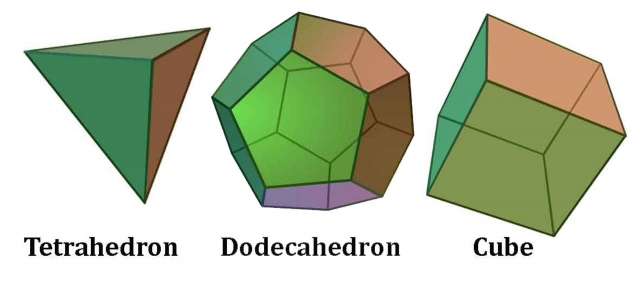Only three types of polygons comprise the faces of the 5 Platonic solids:

Triangle, square, pentagon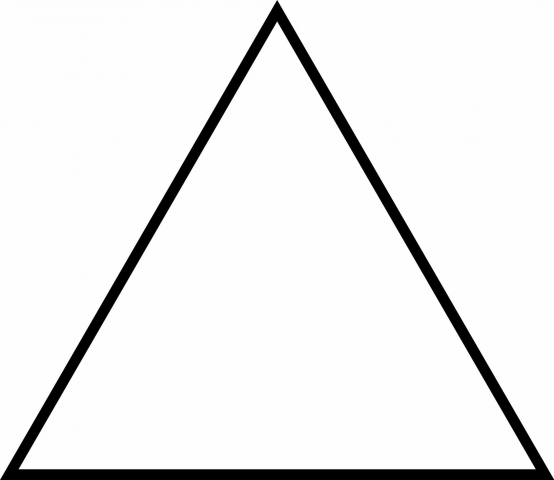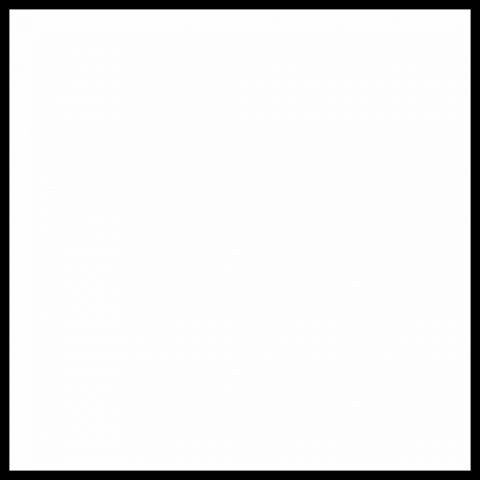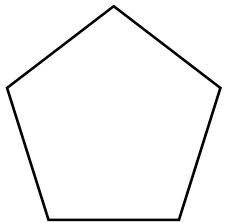There are three mean proportions:

Arithmetic, geometric, harmonic

“In Timaeus, Plato conceived of the geometric series as being the binding force of the universe:

When God put together the body of the universe, he made it of fire and earth. But it is not possible to combine two things properly without a third to act as a bond to hold them together. And the best bond is one that effects the closest unity between itself and the terms it is combining, and this is done by the continued geometrical proportion…”1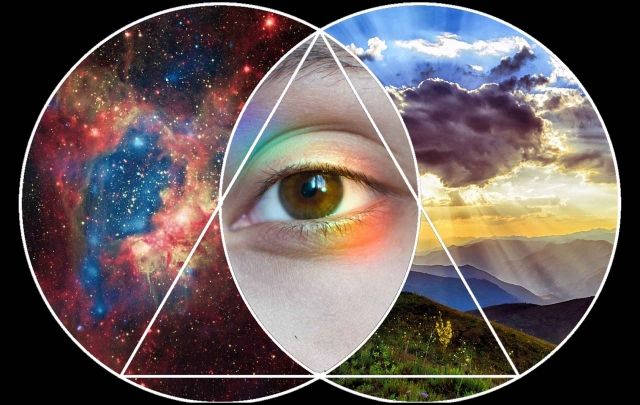“We express the principle of threeness by writing the numeral 3 with a beginning, middle and end.”2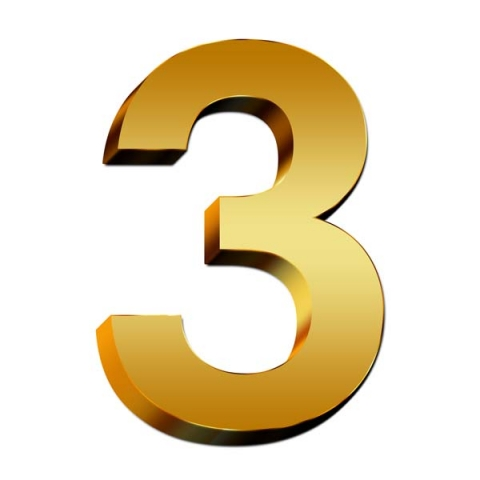That makes three the largest number still written with as many lines as the number represents.

# Powers of Three

32 = 9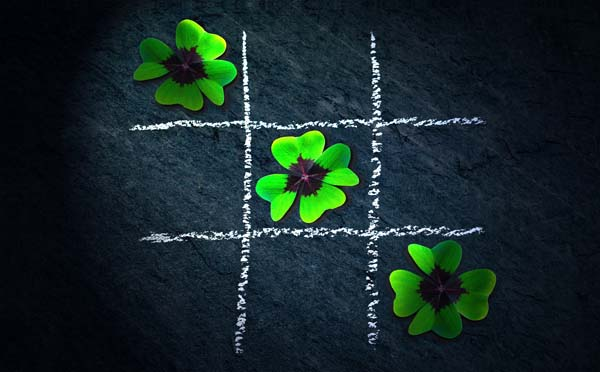33 = 27 = 3 x 9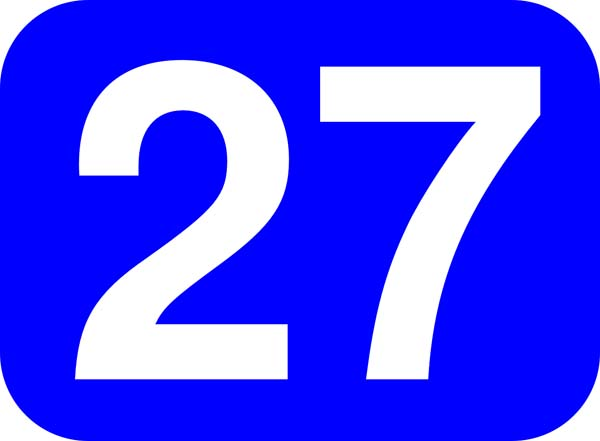27 = 2+3+4+5+6+7

27 is one of few self-locating strings in pi. That means if you start counting with 0, 27 starts at the 27th digit of pi.

27 is the only positive number that is three times the sum of its digits: (2 + 7) x 3 = 27

34=81 = 9 x 9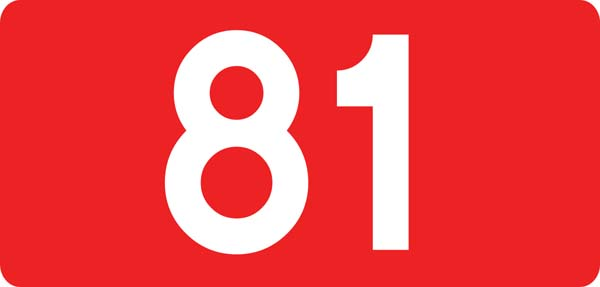1/81 = 0.0123456790123456790123456790123456790…(infinitely repeating decimal of digits in order, except for number 8.)

1:81 = Ratio of the mass of the moon to the mass of Earth.

35=243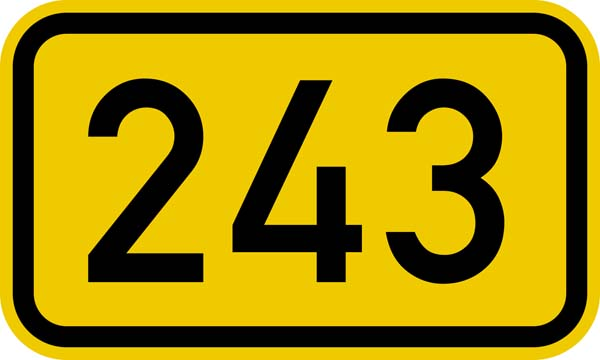243 is the only 3-digit number that is a fifth power (35).

243 is the sum of five consecutive prime numbers (41 + 43 + 47 + 53 + 59)

243 is the number of Earth Days for the planet Venus to complete one Venusian day.

# Theology of Arithmetic – Iamblichus

As we have been doing, we will now take a look at the meaning of the Triad as prepared by Neo-Platonist philosopher, biographer of Pythagoras, Greek mystic and mathematician Iamblichus (245-325 AD).

The triad has a special beauty and fairness beyond all numbers.

It is the first to make actual the potentialities of the monad – oddness, perfection, proportionality, unification, and limit.

It is ‘more than equal’.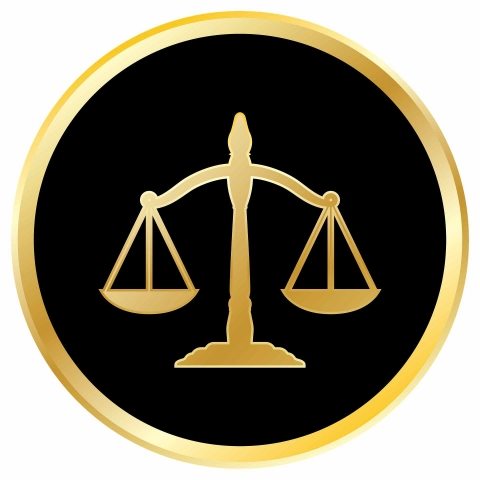It is called ‘mean’ and ‘proportion’.

Three is seen as midway between more and less and has a symmetrical nature.

As we saw above, the Triad is equal to what precedes it, so it gains the specific identity of a mean between the others: (3 = 2 + 1)

On account of the triad, there are three so-called ‘true’ means: arithmetic, geometric and harmonic.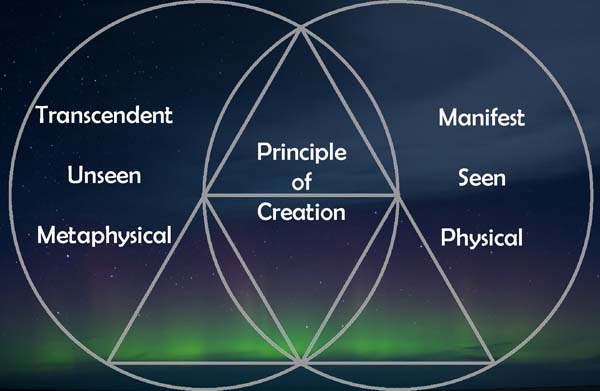Anything in Nature which has process has three boundaries: (beginning, peak (middle) and end (limits) and two intervals: (increase and decrease), with the consequence that the nature of the dyad and ‘either/or’ manifests in the triad by means of its limits.The Triad is called prudence and wisdom – that is, when people act correctly as regards the present, look ahead to the future, and gain experience from what has already happened in the past: so wisdom surveys the three parts of time, and consequently knowledge falls under the triad.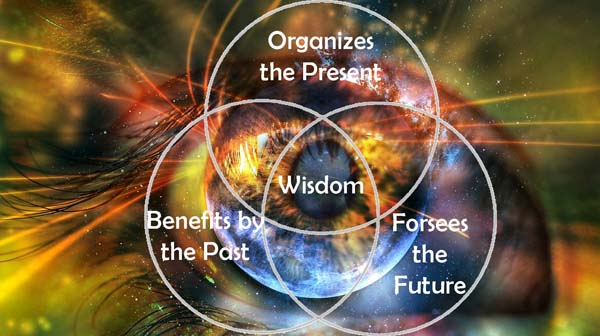The Triad is also called piety.

Among the virtues it is likened to moderation: for it is commensurability between excess and deficiency.Three is the first number to signify totality – beginning, middle and end.

Triangles both reflect and are the first substantiation of the plane.

There are three kinds of triangles – equilateral, isosceles and scalene.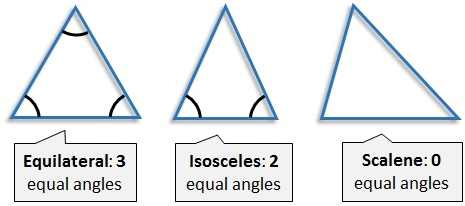There are three rectilinear angles – acute, obtuse, right.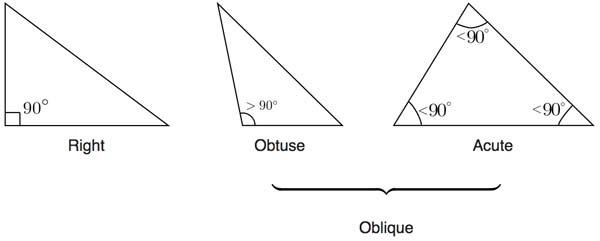There are three parts of time (past, present, future)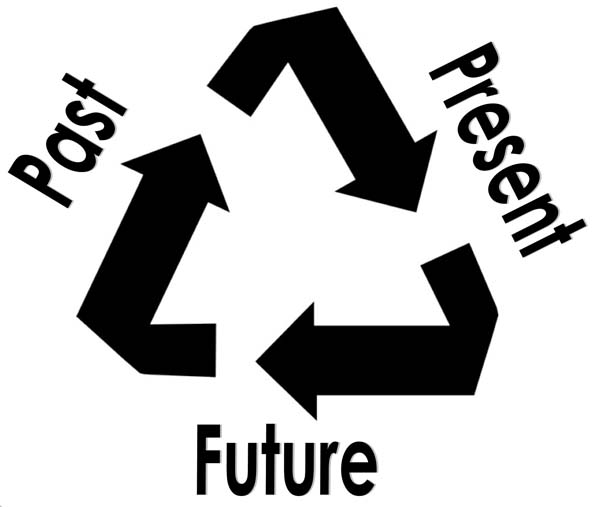three configurations of the moon (waxing, full, waning)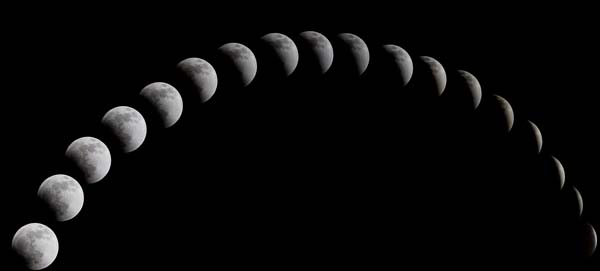three types of irregular motion of the planets (direct, retrogression and stationary)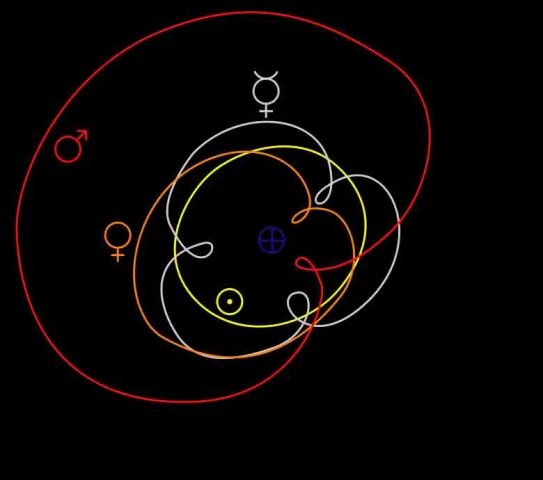three kinds of living creature (land, winged, water)and three Fates in theology, because the whole life of both divine and mortal beings is governed by emission, receiving and thirdly, requital.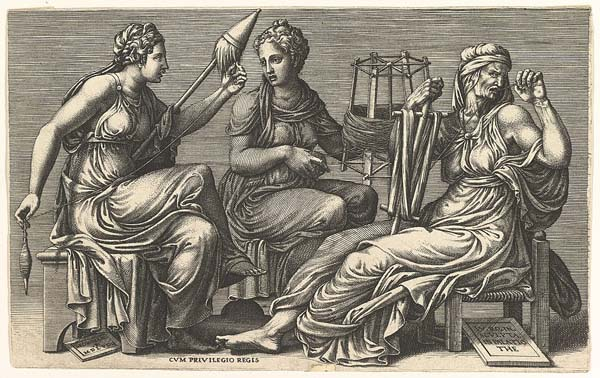The triad gives all things equality and a certain lack of excess and deficiency, having defined and formed matter with the potential for all qualities.

The Triad is considered ‘unyielding’ – that is, not to be worn down; because it is impossible to divide it into two equal parts.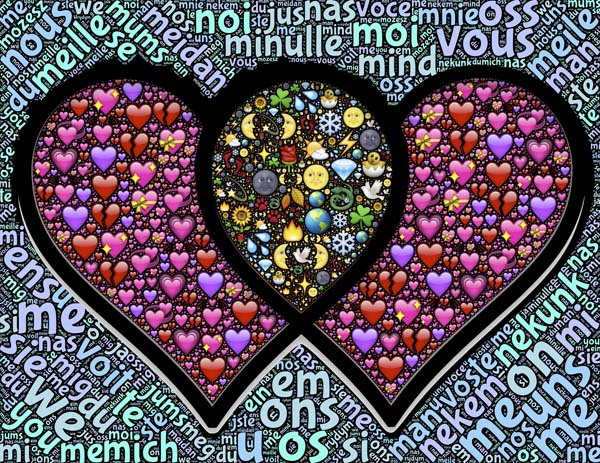It is also called ‘friendship’ and ‘peace’, ‘harmony’, ‘unanimity’ and ‘marriage’: for all these are cohesive and unifying of opposites and dissimilars.# The Tripartite Whole

There seems to be a natural truth in expressing the inherent tripartite wholeness of the universe.

Fewer than three feel incomplete. More than three seems superfluous.

This inclination to divide wholes in triads relates to our sensitivity to archetypes within.

## It is Natural to divide any Whole into Three Parts: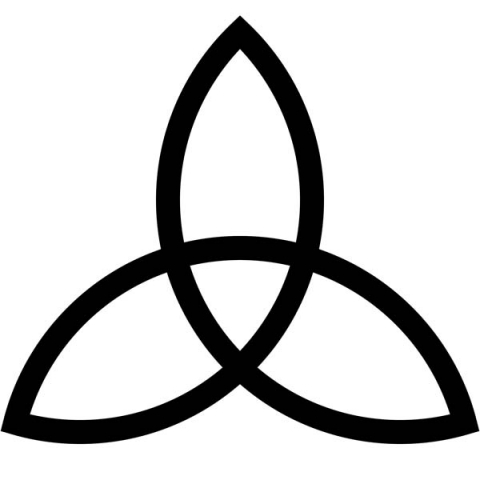• beginning, middle, end
• length, width, height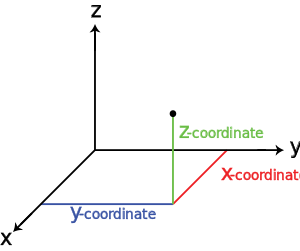• medial, lateral, vertical
• past, present, future
• birth, life, death• dawn, noon, dusk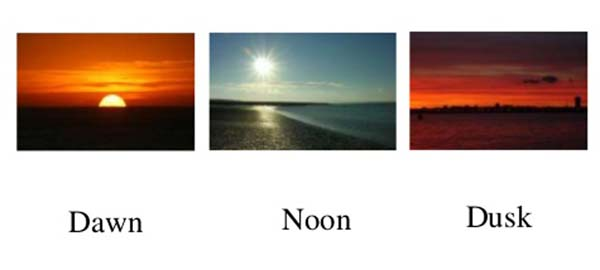• black, white, grey• up, down, middle
• sun, earth, moon• mother, father, child• x, y, z axis
• time, space, matter
• cardinal, fixed, mutable (three modes of astrology)
• input, output, throughput (computer jargon)
• green, yellow, red (traffic lights)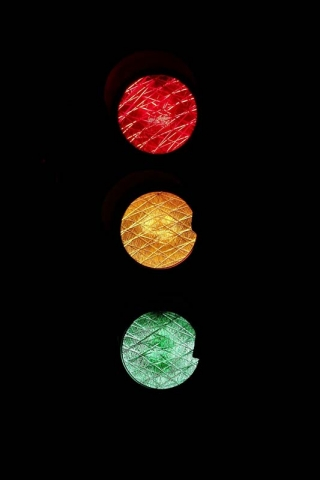• ABC’s

• Bronze, Silver, Gold medals

• first, second, third world countries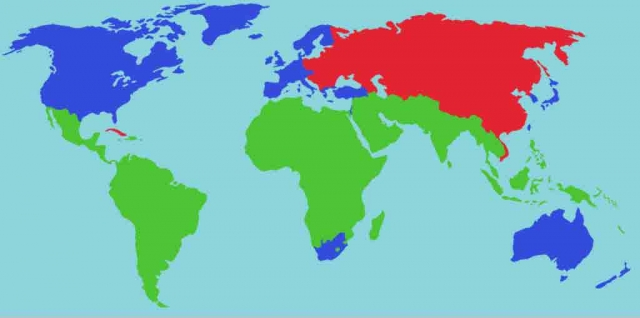• Superlatives: of, relating to, or noting the highest degree of the comparison of adjectives and adverbs:
• good, better, best
• fine, finer, finest
• small, smaller, smallest…etc.
• Number sequences

All sequences known need a minimum of 3 to calculate the entire sequence, with one exception: the Fibonacci Sequence.

• Tripling: 1, 4, 7… (Increases by 3 each time.)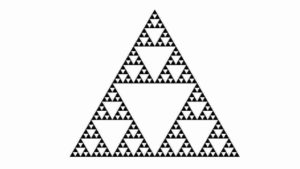Geometric Sequence – made by multiplying the same value (the common ratio) each time.

• Doubling: 2, 4, 8… (Multiplies by 2 each time; 2 is the common ratio.)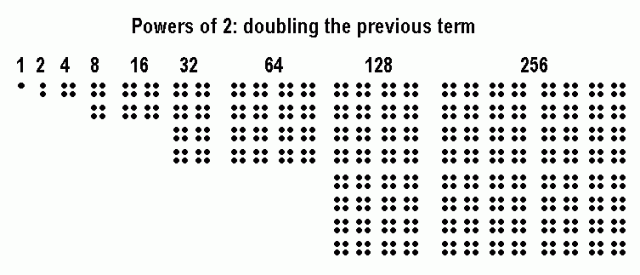Triangular Numbers – generated from a pattern of dots which form a triangle.

• 1, 3, 6, 10, 15, 21, 28, 36, 45, 55, 66, 78, 91, 105…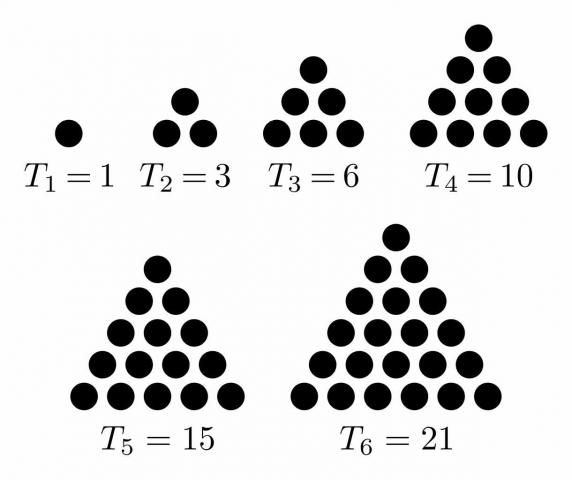Square Numbers – squares of whole numbers.

• 0, 1, 4, 9, 16, 25, 36, 49, 64, 81, 100…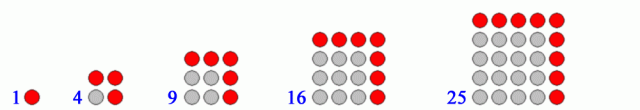Cube Numbers – cubes of integers.

• 1, 8, 27, 64, 125, 216, 343, 512, 729, 1000…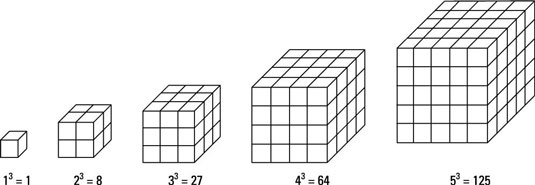Fibonacci Numbers – found by adding the two numbers before it to get the next term.

• 0, 1, 1, 2, 3, 5, 8, 13, 21, 34…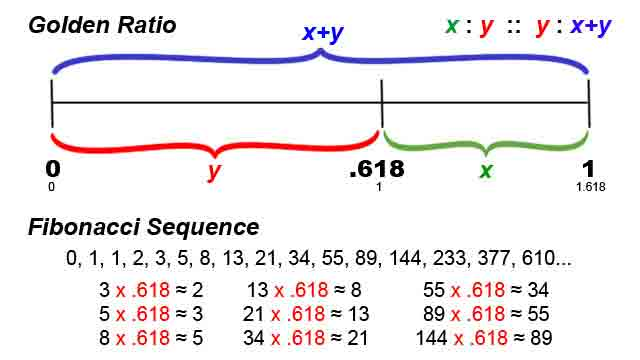Lucas Numbers – similar to Fibonacci numbers; found by adding the two numbers before it.

• 2, 1, 3, 4, 7, 11, 18, 29, 47, 76, 123…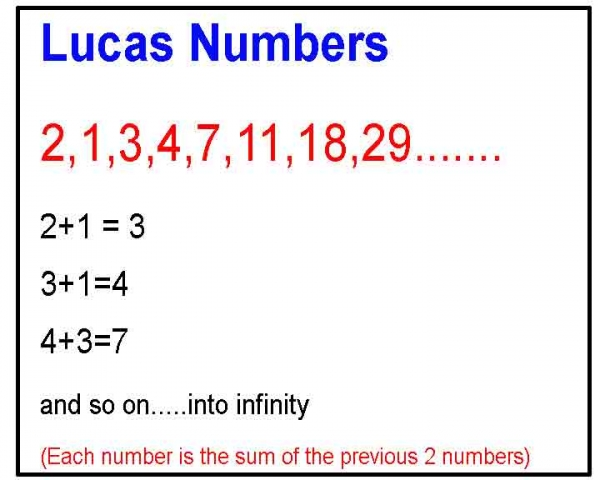1. Kappraff, Jay, Connections: The Geometric Bridge Between Art and Science, World Scientific Publishing Co, 1990
2. Schneider, Michael, A Beginner’s Guide to Constructing the Universe, Harper Perennial, 1994